Courses

# Test: Distance And Speed- 2

## 20 Questions MCQ Test Quantitative Aptitude for GMAT | Test: Distance And Speed- 2

Description
This mock test of Test: Distance And Speed- 2 for GMAT helps you for every GMAT entrance exam. This contains 20 Multiple Choice Questions for GMAT Test: Distance And Speed- 2 (mcq) to study with solutions a complete question bank. The solved questions answers in this Test: Distance And Speed- 2 quiz give you a good mix of easy questions and tough questions. GMAT students definitely take this Test: Distance And Speed- 2 exercise for a better result in the exam. You can find other Test: Distance And Speed- 2 extra questions, long questions & short questions for GMAT on EduRev as well by searching above.
QUESTION: 1

### Rachel drove at a constant speed from inn A along a highway to inn B. Did Rachel reach inn B from inn A in less than an hour? (1) If Rachel had driven to inn C that was 15 kilometers further down the highway, she would have taken 50% more time (2) If Rachel’s average speed for the drive had been 20 kilometers per hour lesser, she would have taken 50% more time

Solution:

Step 1 & 2: Understand Question and Draw Inference

Let distance between Inns A and B = D kilometers (km in short)
Let Rachel’s average speed = S kilometers per hour (kmph in short)
So, Time taken by Rachel to travel from A to B = D/S hours.

To Find: Is D/S < 1 ?

To answer the question, we need to know the value of ratio D/S

Step 3 : Analyze Statement 1 independent

Statement 1 says that: 'If Rachel had driven to inn C that was 15 kilometers further down the highway, she would have taken 50% more time’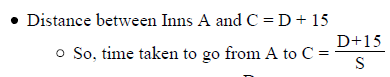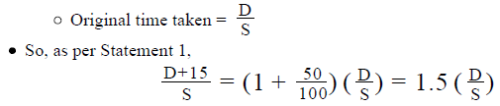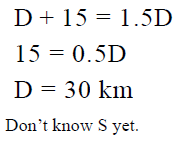So, not sufficient.

Step 4 : Analyze Statement 2 independent

Statement 2 says that: ‘If Rachel’s average speed for the drive had been 20 kilometers per hour lesser, she would have taken 50% more time’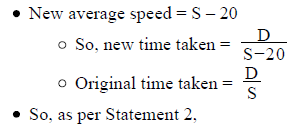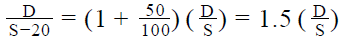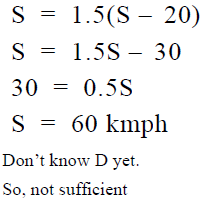Step 5: Analyze Both Statements Together (if needed)

From Statement 1: D = 30 km
From Statement 2: S = 60 kmph
Sufficient to find a unique value of the ratio D/S

QUESTION: 2

### Had Frank driven at an averagespeed of 40 miles per hour, he would have reached his office 10 minutes earlier than he usually did. At what average speed should Frank drive to reach his office 20 minutes earlier than he usually did? (1) Frank usually takes 5/6 hours to reach his office (2) The distance to his office is 80/3  miles

Solution:

Step 1 & 2: Understand Question and Draw Inference

Given:

• Let Frank’s usual time to reach his office be t hours
• So, when speed of Frank = 40 miles per hour, he takes (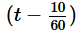) hours to reach his office
• Distance to Frank’s office = 40 * () miles

To Find: Speed of Frank so that he takes (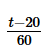) hours to reach his office

• Let the speed of Frank be S miles per hour
• Hence, Distance covered by Frank driving at S miles per hour for() hours = S*()miles
• Now, we know that distance to Frank’s office = 40 * () miles
• So,we can write   S*() =  40 * ()
• Hence, to find the value of S, we need to find the value of t.

Step 3 : Analyze Statement 1 independent

Statement-1: Frank usually takes 5/6  hours to reach his office

• We are given that t = 5/6 hours. So, we can find the value of S

Statement-1 is sufficient to answer the question

Step 4 : Analyze Statement 2 independent

Statement-2: The distance to his office is 80/3 miles

• We know that disatance to Frank’s office is 40 * ()
• So, we can write 40 * () = 80/3 miles .
• As it is a linear equation in t, we can find the value of t
• Once we know the value of t. we can find the value of S

Statement-2 is sufficient to answer the question.

Step 5: Analyze Both Statements Together (if needed)

As we have unique answer from steps 3 and 4 above, this step is not required.

QUESTION: 3

### John leaves from his home at 10 AM and starts driving towards a city that is 300 kilometres away, at a constant speed of 50 miles per hour. His brother Martin leaves the home at 11:30 AM and starts driving on the same route at a constant speed of 60 miles per hour. If they stop driving once they reach the city, which of the following statements must be true? (1 mile = 1.6 kilometres) I. John reaches the city before Martin II. At 1:30 PM, John is 55 kilometres ahead of Martin III. Martin overtake John at 4 PM

Solution:

Given:

• Home – City distance = 300 km
• John:
• Speed = 50 miles per hour (Note that the distance is given in kilometers and John’s speed is given in miles per hour)
• 1 mile = 1.6 kilometers
• Time of start= 10 AM
• Martin:
• Speed = 60 miles per hour
• Time of start = 11:30 AM

To Find: Which of the 3 statements must be true?

Approach:

1. We will evaluate the 3 statements one by one

Working out:

• Evaluating Statement I
• To evaluate this statement, we need to find the time at which John reaches the city and the time at which Martin reaches the city
• John:
• Speed = 50 miles per hour = 50*1.6 kilometers per hour
• So, time (hours) =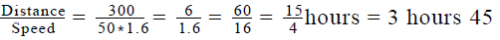• John starts at 10 AM
• So, John reaches the city at 10 AM + 3 hours 45 min = 1:45 PM
• Martin:
• Speed = 60 miles per hour = 60*1.6 kilometers per hour
• So, time (hours) =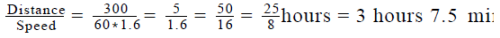• Martin starts at 11:30 AM
• So, Martin reaches the city at 11:30 AM + 3 hours 7.5 min = 2: (37.5) PM
• Therefore, Statement I is indeed true
• Evaluating Statement II
• At 1:30 PM, John has been travelling for (1:30 PM – 10 AM =) 3.5 hours and Martin for (1:30 PM – 11:30 AM =) 2 hours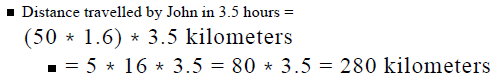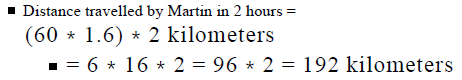• So, distance between them at 1:30 PM = 280 – 192 = 88 km
• Therefore, Statement II is not true

• Evaluating Statement III
• In our analysis of Statement I, we’ve already seen that John reached the city before Martin. Since both of them stop driving once they reach the city, Martin never manages to overtake John (and none of them are still driving at 4 PM)
• Therefore, Statement III is not true.
• Thus, we see that out of the 3 statements, only Statement I is true.

Looking at the answer choices, we see that the correct answer is Option A

QUESTION: 4

Raymond travelled from city A to city C via city B. Was his average speed for the journey from city A to city B greater than his average speed for the journey from city B to city C?

(1) Raymond’s average speed of the journey from city A to city B was greater than his average speed of the journey from city A to city C.

(2) Had Raymond travelled at a constant speed from city A to city C, the time taken by him to travel from city A to city B would have been lesser than the time taken by him to travel from city B to city C.

Solution:

Step 1 & 2: Understand Question and Draw Inference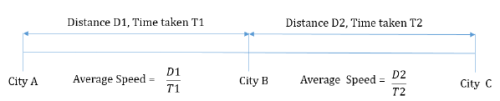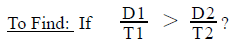Step 3 : Analyze Statement 1 independent

(1) Raymond’s average speed of the journey from city A to city B was greater than his average speed of the journey from city A to city C.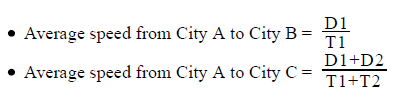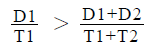As the denominators of the inequalities are positive, we can multiply and solve it to get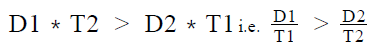Step 4 : Analyze Statement 2 independent

(2) Had Raymond travelled at a constant speed from city A to city C, the time taken by him to travel from city A to city B would have been lesser than the time taken by him to travel from city B to city C.
Let Raymond’s constant speed be S kilometeres per hour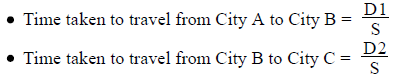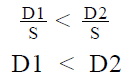It does not tell us anything about the average speeds of Raymomd from city A to city B and city B to city C. Insufficient to answer

Step 5: Analyze Both Statements Together (if needed)

Since, we have a unique answer from step 3, this step is not required.

QUESTION: 5

Two siblings Mark and Steve start from the same point and walk in the same direction at speeds of 5 kilometres per hour and 10 kilometres per hour respectively. After walking for an hour, Mark turns around and walks back along the same path to the starting point. Mark rests for half an hour at the starting point, and then hires a taxi driving at 30 kilometres per hour to catch up with Steve. If the taxi charges \$10 for the first 2 kilometres and \$2 for every subsequent 500 metres, how much in dollars does Mark pay for the taxi ride?

Solution:

Given:

• Speed of Mark = 5 kilometres per hour
• Speed of Steve = 10 kilometres per hour
• Time after which Mark turns around and walks back = 1 hour
• Time for which Mark rests = 0.5 hour
• Speed of taxi = 30 kilometres per hour
• Charges for the first 2 kilometres = \$10
• Chrages for every subsequent 500 metres = \$2

To Find: Amount that Mark paid to the taxi driver?

Approach:

1. For finding the amount that Mark paid to the taxi driver, we need to find the distance travelled by the taxi.
2. As the taxi started from the starting point of Steve and stopped when it caught up with Steve, the (Distance travelled by the taxi) = (Distance travelled by Steve since he started walking)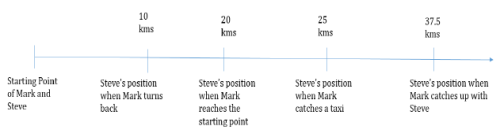3. Distance travelled by Steve = Speed of Steve * Time taken
• We are given that speed of steve = 10 kilometres per hour. We need to find the time for which Steve travelled
4. The travelling time of Steve can be divided into 4 phases:
1. Phase-I: Time for which Mark and Steve both walked = 1 hour
2. Phase-II: Time taken by Mark to reach back the starting point = 1hour
• As Mark travelled one way for 1 hour and travelled back the same path at the same speed, he will take the same time
3. Phase-III: Time for which Mark rests = 0.5 hour
• Plesae note that Steve kept walking ahead during this time
4. Phase-IV: Time for which the taxi drove = t hours
1. For this phase, the distance travelled by taxi = total distance travelled by Steve
2. The time for which the taxi would travel would be equal to the time for which Steve would be travelling in this phase.
3. As we know the speeds of both taxi and Steve, we can formulate an equation by equating the time taken.
5. We will find the distance travelled by Steve in each of the phases and then sum it up to get the total distance distance travelled by Steve

Working out:

1. Phase-I: Distance travelled by Steve = 10 * 1 = 10 kilometres
2. Phase-II: Distance travelled by Steve = 10 * 1 = 10 kilomtres
3. Phase-III: Disatnce travelled by Steve = 10 * 0.5 = 5 kilomtres
4. Phase-IV:
• Let the distance covered by Steve in time t hours be x kilometres.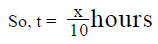• Total distance covered by taxi to catch up with Steve = 10 + 10 + 5 + x = 25 + x kilometres
• Time taken by taxi to cover 25 + x kilometres, i.e. t =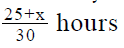• We can equate the time taken by taxi and Steve
•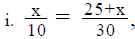5. So, total distance travelled by the taxi = 25 + 12.5 = 37.5 kilomtres
6. Charges for the first 2 kilometres = \$10
7. Charges for the rest 35.5 kilomtres = 35.5 * 2 * 2 = \$142
8. Hence, total amount paid to the taxi driver = \$10 + \$ 142 = \$152.

QUESTION: 6

Robert and Timothy both left their respective homes at 1 PM. Robert walked till a lake that was between 2.5 kilometres and 3 kilometres from his home, inclusive, and returned home between 1:55 PM and 2:05 PM. If Timothy walked 5 kilometres more than Robert and returned to her home at 3 PM, which of the following statements must be true?

I. The walking speed of Timothy was greater than that of Robert

II. Robert’s walking speed was close to 3 kilometers per hour

III. Had Robert covered 5 more meters every 18 seconds, his walking speed would have been greater than Timothy’s

Solution:

Given:

• Robert
• Let the distance covered be DR and time taken be TR
• 2.5*2 km ≤ DR ≤ 3*2 (distance in round trip is double the one-way distance)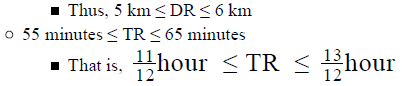• Timothy
• Let the distance covered be DT and time taken be TT
• As Timothy walked 5 kilometers more than Robert, she would have walked a minimum distance of 5 + 5 = 10 kms and a maximum distance of 6 + 5 = 11 kms.
• So, 10 km ≤ DT ≤ 11
• TT = 2 hours

To Find:

Let Robert’s speed be SR and Timothy’s speed be ST

ST > SR?
SR ∼ 3 kmph?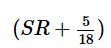meters per second (in short  mps) > ST ?

Approach:

• Find range of SR and ST (since definite values of DR, TR and TT are not given, definite values of SR and ST cannot be found)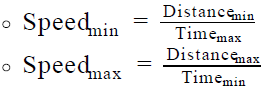• Check the validity of the 3 statements

Working out: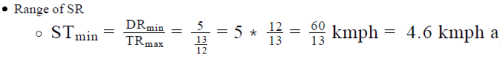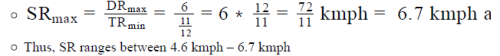• Range of ST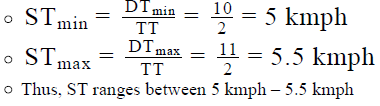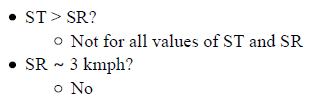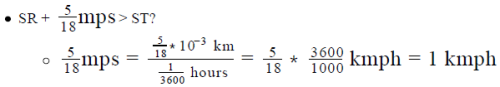• SR + 1 kmph: 5.6 kmph – 7.7 kmph
• Thus, Robert’s speed would definitely have been greater than
• Timothy’s
• So, Yes

Thus, only St. III is a must be true statement.

QUESTION: 7

Brian drives a certain part of his journey at an average speed of 30 miles per hour and the rest at an average speed of 60 miles per hour. If he drives for 100 miles, is his average speed for the journey greater than 45 miles per hour?

(1) Brian drives for 30 miles per hour for twice the time than he drives for 60 miles per hour.

(2) Had Brian driven at an average speed of 40 miles per hour rather than 30 miles per hour, his average speed for the
journey would have been 48 miles per hour.

Solution:

Step 1 & 2: Understand Question and Draw Inference

• Let the distance for which he drives for 30 miles per hour be x miles.
• Distance for which he drives for 60 miles per hour = 100 – x miles
•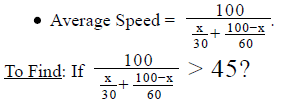Step 3 : Analyze Statement 1 independent

(1) Brian drives for 30 miles per hour for twice the time than he drives for 60 miles per hour.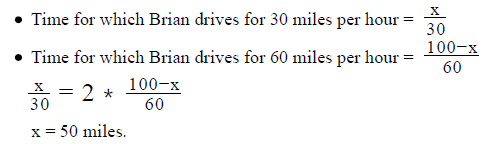As we know the values of x, we can find if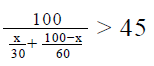Step 4 : Analyze Statement 2 independent

(2) Had Brian driven at an average speed of 40 miles per hour rather than 30 miles per hour, his average speed for the journey would have been 48 miles per hour.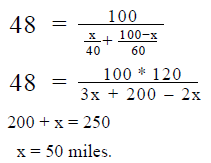As we know the values of x, we can find if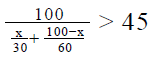Step 5: Analyze Both Statements Together (if needed)

Since, we have a unique answers from steps 3 and 4, this step is not needed.

QUESTION: 8

An international flight takes off from New Delhi in India for Washington DC in the United States of America via Doha, where the flight stops for 2 hours and 40 minutes. During flight, the average speed of the plane is 660 kilometres per hour and the flight reaches Washington DC, at 3:50 PM, Washington time. If the distance between New Delhi and Washington DC via Doha is 12000 kilometres and New Delhi is 9 hour 30 minutes ahead of Washington DC, what is the approximate time in New Delhi when the flight takes off from there?

Solution:

Given:

• Delhi -> Doha (2 hr 40 min stop) -> DC
• Average flying speed = 660 kmph
• Flying distance = 12000 km
• DC Time at Arrival in DC = 3:50 PM
• Delhi Time – DC Time = 9.5 hours

To Find:

Delhi time at take-off

Approach:

Delhi time at take – off = Delhi time at arrival in DC – Total journey time
of the plane
Delhi time at arrival in DC = DC Time at arrival in DC + 9.5 hours
Total journey time of the plane = Flying Time + Stopover time at Doha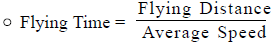Working out:

Delhi Time at arrival in DC = 3:50 PM + 9.5 hours = 1:20 AM
Flying Time :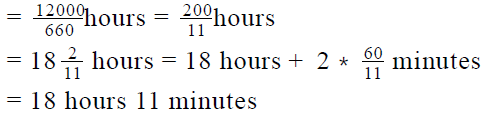• Total Journey time of the plane = 18 hours 11 minutes + 2 hours 40 minutes = 20 hours 51 minutes
• So, Delhi time at take – off = 1:20 AM – 20 hours 51 minutes

= 1:20 AM – (24 hours – 3 hours 9 min)
= (1:20 AM – 24 hours) + 3 hour 9 min
= 1:20 AM + 3 hours 9 min
= 4:29 AM
Since this is the closest to 4:30 AM, the correct answer is Option B.

QUESTION: 9

On a trip of 60 kilometres, Rita covered the first 15 kilometres at an average speed of 75 kilometres per hour and the following 35 kilometres at an average speed of 140 kilometres per hour. At what average speed did she cover the last part of her trip if her average speed for the whole trip was 80 kilometres per hour?

Solution:

Given:

• The Whole Trip:
• Total distance = 60 km
• The Average Speed for the whole trip = 80 kmph
• Part 1 of Trip:
• Distance = 15 km
• Average Speed = 75 kmph
• Part 2 of Trip:
• Distance = 35 km
• Average Speed = 140 kmph
• The Last Part of Trip:
• Distance = 60 – (15 + 35) = 60 – 50 = 10 km

To Find: Average Speed for The Last Part of Trip

Approach: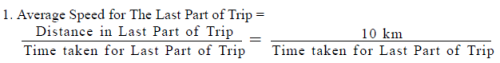2. So, to answer the question, we need to find Time Taken for Last Part of Trip: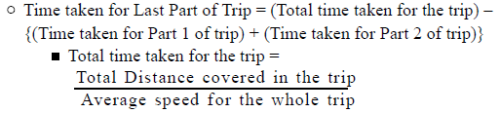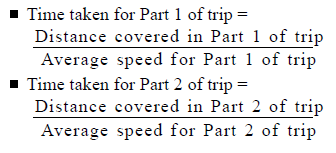Working out:

• Finding Time taken for Last Part of Trip: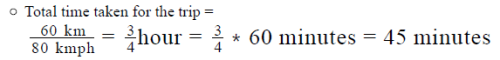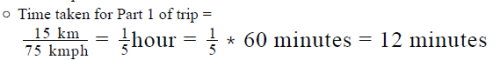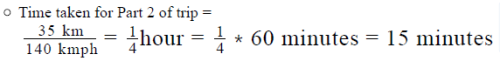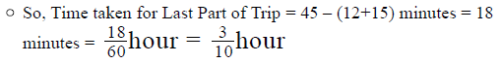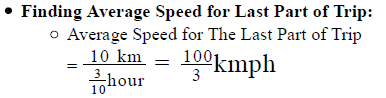Looking at the answer choices, we see that the correct answer is Option D

QUESTION: 10

A boat travels from Point A to Point B in the direction of water and then returns to Point A. In still water, the boat travels at a
speed of 28 kilometres per hour. If the speed of the water current is 4 kilometres per hour and the time taken for the round-trip is 2
hours and 55 minutes, what is the total distance travelled by the boat in the round trip?

Solution:
QUESTION: 11

At 11 AM, Abraham leaves from his home for a meeting that is scheduled for 1 PM. If the venue of the meeting is not less than 100 kilometers away and Abraham drives at a speed not less than 60 kilometers per hour, will he be late for the meeting?

(1) The venue of the meeting is less than 120 kilometers away

(2) He does not drive at a speed greater than 80 kilometers per hour

Solution:
QUESTION: 12

A marathon runner starts the 26 mile run at 7.30 AM and reaches the finish line at 9.45 AM. If he runs at a constant speed throughout the marathon and water stations are put up along the route of the marathon after every 500 metres, which of the following is the closest to the time taken by the runner to go from one water station to the next one? (1 mile = 1.6 kilometres)

Solution:
QUESTION: 13

Megan and David start running along the perimeter of a square park. To make one round of the park, Megan takes 1 minute and
David takes 1 minute and 36 seconds. If both Megan and David burn 24 calories in making one round of the park, in how much
time will Megan have burnt 120 calories more than David?

Solution:
QUESTION: 14

Two trains start from City A and travel towards City B, which is at a distance of 100 miles from City A, at the same time with an
average speeds of 60 miles per hour and 80 miles per hour respectively. A train starts from City B at the same time and travels towards City A at an average speed of 70 miles per hour. How far from city B is the slower train starting from City A when the faster train starting from City A meets the train starting from City B?

Solution:
QUESTION: 15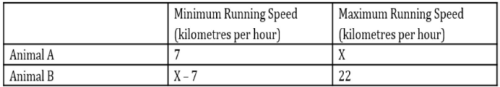The table above shows the minimum and the maximum running speeds of two animals. If Animal A runs between two poles that
are placed 20 metres apart in 3 seconds and Animal B runs between the same poles in 4 seconds, which of the following
cannot be the value of X?

I. 23
II. 26
III. 27

Solution:
QUESTION: 16

Patrick takes 2 hours to drive in his car from point A to point B. What is the distance between A and B?

(1) If his average speed for the journey had been 20 kilometers per hour greater, he would have taken only 1 hour and 36 minutes to reach point B

(2) Patrick covered an average of 800 meters every 36 seconds

Solution:
QUESTION: 17

James started from his home and drove eastwards at a constant speed. Exactly 90 minutes after James stated from his home, his brother Patrick started from the same point and drove in the same direction as James did at a different constant speed. Patrick overtook James exactly 90 minutes after Patrick started his journey and then continued driving at the same speed for another 2 hours. By what percentage should Patrick reduce his speed so that James could catch up with Patrick in exactly 8 hours after Patrick overtook James?

Solution:
QUESTION: 18

Alex and Hales started rowing towards one another at constant speeds and met after rowing for an hour. The initial distance between Alex and Hales was 20 kilometers and Alex rowed downstream while Hales rowed upstream during this journey. On some other day, the time taken by Alex alone, rowing at the same constant speed, to cover the distance between these two points going upstream was 75 minutes more than the time taken by him to cover the same distance going downstream. What was the time taken in hours by Hales to cover 20 kilometers going upstream? Assume the speed of the stream to be 4 kilometers per hour

Solution:
QUESTION: 19

On a Monday, John left from his home for his office at 09:05 AM and reached his office at 10:00 AM. On Tuesday, he left from his home for his office 15 minutes later than the time he left his home on Monday and reached his office 4 minutes later than the time he reached his office on Monday. If on Tuesday, John drove at an average speed that was 15 kilometres per hour faster than his average speed on Monday, how far in kilometres was his office from his home?

Solution:
QUESTION: 20

A man starts rowing from Point A in the direction of the water flow at a constant speed of 10 kilometers per hour. After rowing for 10 kilometers, he remembers that he forgot an important object at Point A and turns back to get it. After getting the object, he starts moving again in the direction of water flow, but due to an onset of exhaustion, he now rows at a constant speed of 8 kilometers per hour. After rowing for 26 kilometers, he reaches Point D, where he rests for 1 hour, and then resumes his journey in the same direction, rowing at a constant speed of 9 kilometers per hour, till he reaches Point B, which is 14 kilometers downstream from Point D. If the speed of water flow is 5 kilometers per hour, what is the average speed of the man for the entire journey?

Solution: